### Home > MC2 > Chapter 10 > Lesson 10.1.4 > Problem10-48

10-48.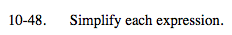6 · 6 · 6 · 6 · 6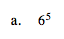$\frac{2}{3}\cdot\frac{2}{3}\cdot\frac{2}{3}$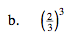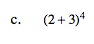Following the order of operations, first simplify the terms in parentheses.

(5)4

Then simplify the terms with exponents.

5 · 5 · 5 · 5

625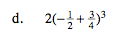$\frac{1}{32}$